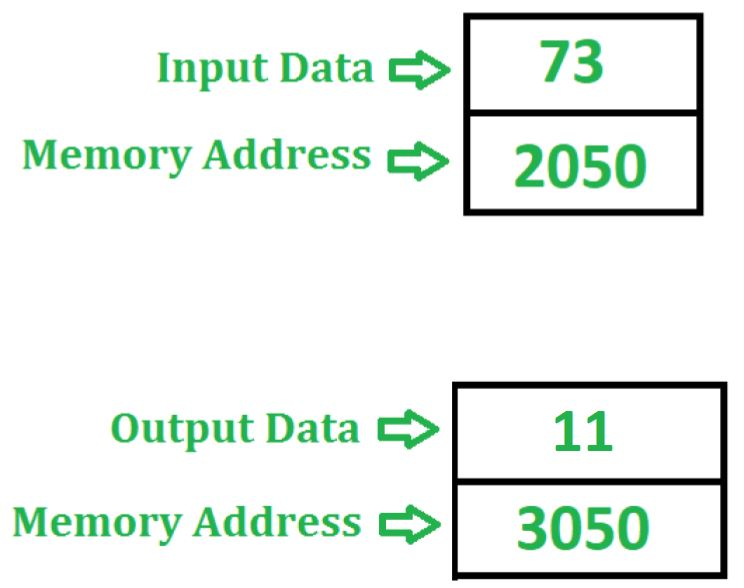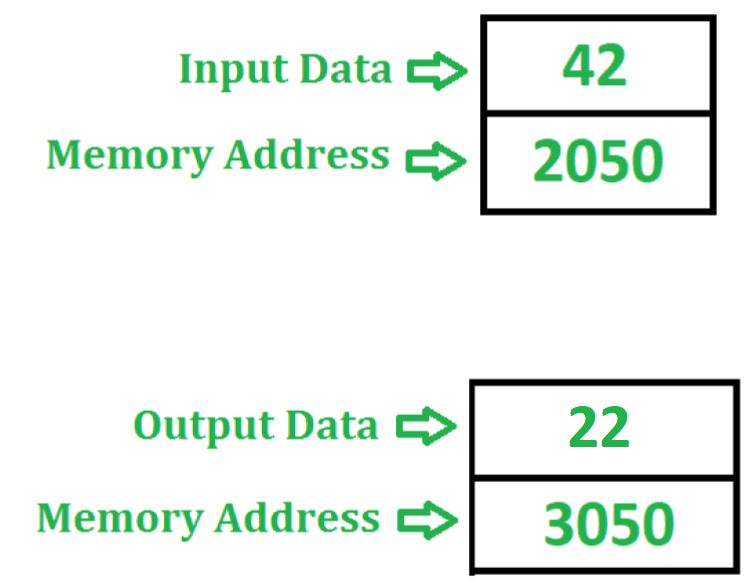# 8085 program to check whether the given number is even or odd

Problem – Write an assembly language program in 8085 microprocessor to check whether the 8 bit number which is stored at memory location 2050 is even or odd. If even, store 22 at memory location 3050 otherwise store 11 at memory location 3050.

Example –A number is said to be odd if its lower bit is 1 otherwise even. Therefore to identify whether the number is even or odd, we perform AND operation with 01 by the help of ANI instruction. If number is odd then we will get 01 otherwise 00 in accumulator. ANI instruction also affect the flags of 8085. Therefore if accumulator contains 00 then zero flag becomes set otherwise reset.

Algorithm –

1. Load the content of memory location 2050 in accumulator A.
2. Perform AND operation with 01 in value of accumulator A by the help of ANI instruction.
3. Check if zero flag is set, i.e if ZF = 1 then store 22 in accumulator A otherwise store 11 in A.
4. Store the value of A in memory location 3050

Program –

2000 LDA 2050 A <- M
2003 ANI 01 A <- A (AND) 01
2005 JZ 200D Jump if ZF = 1
2008 MVI A 11 A <- 11
200D MVI A 22 A <- 22
200F STA 3050 M <- A
2012 HLT END

Explanation – Registers used A:

1. LDA 2050 –loads the content of memory location 2050 in accumulator A
2. ANI 01 –performs AND operation between accumulator A and 01 and store the result in A
3. JZ 200D –jump to memory location 200D if ZF = 1
4. MVI A 11 –assign 11 to accumulator